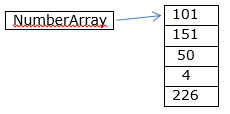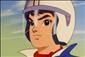# Arrays in Java: Part 1

Arrays in Java: Part 1

We create arrays when we want to operate on a collection of variables of the same data type or pass them all around together. An array is essentially a collection of elements of the same data type. The elements are also called components of the array. Each element of the array is accessed using a unique index value, also called a subscript. For example, an array of integers contains several elements, each of type int, and an array of floating-point numbers contains several elements, each of type float. Because array elements are accessed using a single variable name coupled with a subscript, we do not need to create several unique variable names in our program code to store and access many variables having the same data type.The array shown here have five integer variables. Each variable will be accessed using the single name numbers, along with a unique index in the array. Each variable will hold an integer value that is independent of the values held by other elements. Each element can be accessed and modified individually..

## Declaring an Array:

The general syntax for declaring an array is as follows:

type arrayName[ ];

But, we can also declare an array as follows:

type [ ] arrayName;

The square brackets in this syntax are also called the indexing operator because they specify the index of an element in an array. The type specifies the type of element that the array is going to store. The arrayName specifies the name by which the elements of the array are addressed in the program code. Note that like in many other languages, the declaration does not allow us to specify the size of the array.

Int [ ] integerNumbers;

The name of the array in this declaration is integerNumbers. Thus, each element of the array would be accessed using this name along with an appropriate index value.

## Creating Arrays

Now that we know how to declare an array variable, the next task is to allocate space for the array elements. To allocate space, we use new keyword. For example, to create an array of 10 integers, we would use the following code:

Int [ ] integeNumbers;

integerNumbers = new int;

The first statement declares a variable called numbers of the array type, with each element of type int.

The second statement allocates contiguous memory for holding 10 integers and assigns the memory address of the first element to the variable integerrNumbers. An array initializer provides initial values for all its components. We may assign different values to these variables somewhere in our code.

## Accessing and Modifying Array Elements

Now that we know how to create an array, our next task is to access the array elements. We use an index to access the elements of an array. Each element of the array has a unique index value. An element of an array is accessed by using the array name followed by its index value written in square brackets. The general syntax of accessing an array element is as follows:

arrayName [ indexValue ] ;

For example, consider the following declaration of an array of integers:

int[ ] numbers = new int ;

Note how the declaration and allocation are done in the same program statement. In this declaration, there is a total of five elements in the numberArray array. The array index starts with a value of zero. Thus, the first element of the array is accessed using the following syntax:

numbers

Last updated:9/7/2019 12:16:18 AM#### David Miller